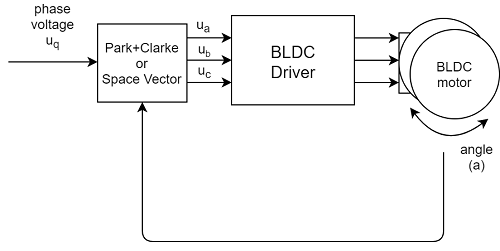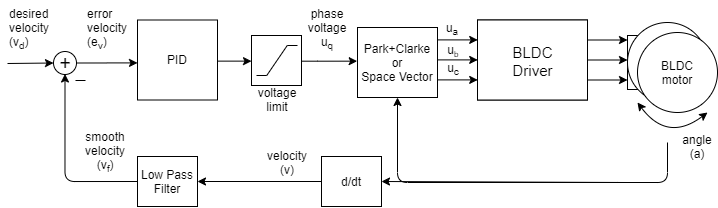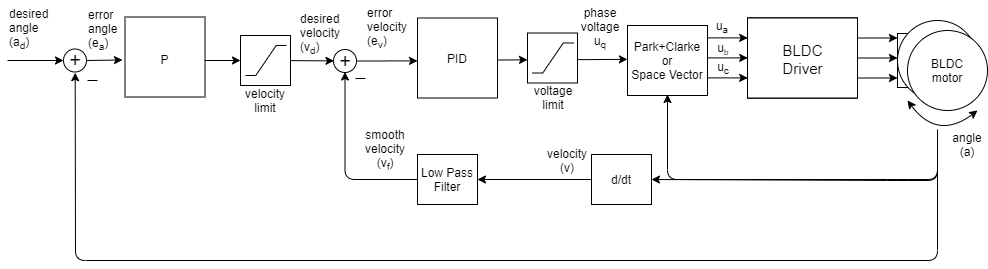# Motion control implementation v1.6

SimpleFOClibrary implements 3 motion control loops:

• Torque control using voltage
• Velocity motion control
• Position/angle control

The motion control algorithm is chosen by seting the motor.controller variables with one of the ControlType structure:

// Motion control type
enum ControlType{
voltage,// Torque control using voltage
velocity,// Velocity motion control
angle// Position/angle motion control
};


This will look like:

motor.controller = ControlType::voltage;
// or
motor.controller = ControlType::velocity;
// or
motor.controller = ControlType::angle;


This variable can be changed in real-time as well!

## Real-time execution move()

The real-time motion control is executed inside move() function. Move function receives executes one of the control loops based on the controller variable. The parameter new_target of the move() function is the target value so be set to the control loop. The new_target value is optional and doesn’t need to be set. If it is not set the motion control will use motor.target variable.

Here is the implementation:

// Iterative function running outer loop of the FOC algorithm
// Behavior of this function is determined by the motor.controller variable
// It runs either angle, velocity or voltage loop
// - needs to be called iteratively it is asynchronous function
// - if target is not set it uses motor.target value
void BLDCMotor::move(float new_target = NOT_SET) {
// check if target received through the parameter new_target
// if not use the internal target variable (motor.target)
if( new_target != NOT_SET ) target = new_target;
// get angular velocity
shaft_velocity = shaftVelocity();
// choose control loop
switch (controller) {
case ControlType::voltage:
// set the target voltage for FCO loop
voltage_q =  target;
break;
case ControlType::angle:
// angle set point
shaft_angle_sp = target;
// calculate the necessary velocity to achieve target position
shaft_velocity_sp = positionP( shaft_angle_sp - shaft_angle );
// calculate necessary voltage to be set by FOC loop
voltage_q = velocityPI(shaft_velocity_sp - shaft_velocity);
break;
case ControlType::velocity:
// velocity set point
shaft_velocity_sp = target;
// calculate necessary voltage to be set by FOC loop
voltage_q = velocityPI(shaft_velocity_sp - shaft_velocity);
break;
}
}


## Shaft velocity filtering shaftVelocity

The first step for velocity motion control is the getting the velocity value from sensor. Because some sensors are very noisy and especially since in most cases velocity value is calculated by derivating the position value we have implemented a Low Pass filter velocity filter to smooth out the measurement. The velocity calculating function is shaftVelocity() with implementation:

// shaft velocity calculation
float BLDCMotor::shaftVelocity() {
float Ts = (_micros() - LPF_velocity.timestamp) * 1e-6;
// quick fix for strange cases (micros overflow)
if(Ts <= 0 || Ts > 0.5) Ts = 1e-3;
// calculate the filtering
float alpha = LPF_velocity.Tf/(LPF_velocity.Tf + Ts);
float vel = alpha*LPF_velocity.prev + (1-alpha)*sensor->getVelocity();
// save the variables
LPF_velocity.prev = vel;
LPF_velocity.timestamp = _micros();
return vel;
}


The low pass filter is standard first order low pass filter with one time constant Tf which is configured with motor.LPF_velocitystructure:

// Low pass filter structure
struct LPF_s{
float Tf; // Low pass filter time constant
long timestamp; // Last execution timestamp
float prev; // filtered value in previous execution step
};


## Torque control using voltage

Since for most of the low-cost gimbal motors and drivers current measurement is usually not available then the torque control has to be done using voltage directly.This control loop makes an assumption that the voltage is proportional to the current which is proportional to the torque. Which is in general correct but not always. But in broad terms, it works pretty well for low current applications (gimbal motors). This is the same assumption we usually make with DC motors.

The control loop has trivial implementation, basically set the target voltage to the voltage_q variable in order to be set to the motor using the FOC algorithm loopFOC().

API usage

## Velocity motion control

Once we have the current velocity value and and the target value we would like to achieve, we need to calculate the appropriate voltage value to be set to the motor in order to follow the target value.And that is done by using PI controller in velocityPI() function.

// velocity control loop PI controller
float BLDCMotor::velocityPI(float tracking_error) {
return controllerPI(tracking_error, PID_velocity);
}


The BLDCMotor class has implemented generic PI controller function called controllerPI().

// PI controller function
float BLDCMotor::controllerPI(float tracking_error, PI_s& cont){
float Ts = (_micros() - cont.timestamp) * 1e-6;

// quick fix for strange cases (micros overflow)
if(Ts <= 0 || Ts > 0.5) Ts = 1e-3;

// u(s) = (P + I/s)e(s)
// Tustin transform of the PI controller ( a bit optimized )
// uk = uk_1  + (I*Ts/2 + P)*ek + (I*Ts/2 - P)*ek_1
float tmp = cont.I*Ts*0.5;
float voltage = cont.voltage_prev + (tmp + cont.P) * tracking_error + (tmp - cont.P) * cont.tracking_error_prev;

// antiwindup - limit the output voltage_q
if (abs(voltage) > cont.voltage_limit) voltage = voltage > 0 ? cont.voltage_limit : -cont.voltage_limit;
// limit the acceleration by ramping the the voltage
float d_voltage = voltage - cont.voltage_prev;
if (abs(d_voltage)/Ts > cont.voltage_ramp) voltage = d_voltage > 0 ? cont.voltage_prev + cont.voltage_ramp*Ts : cont.voltage_prev - cont.voltage_ramp*Ts;

cont.voltage_prev = voltage;
cont.tracking_error_prev = tracking_error;
cont.timestamp = _micros();
return voltage;
}


The PI controller is configured with motor.PID_velocity structure:

// PI controller configuration structure
struct PI_s{
float P; // Proportional gain
float I; // Integral gain
float voltage_limit; // Voltage limit of the controller output
float voltage_ramp;  // Maximum speed of change of the output value
long timestamp;  // Last execution timestamp
float voltage_prev;  // last controller output value
float tracking_error_prev;  // last tracking error value
};


API usage

## Position motion control

Now when we have the velocity control loop explained we can build our position control loop in cascade as shown on the image.When we have target angle we want to achieve, we will use the P controller to calculate necessary velocity we need and then the velocity loop will calculate the necessary voltage votage_q to achieve both velocity and angle that we want.

The position P controller is implemented in positionP() function:

// P controller for position control loop
float BLDCMotor::positionP(float ek) {
// calculate the target velocity from the position error
float velocity_target = P_angle.P * ek;
// constrain velocity target value
if (abs(velocity_target) > velocity_limit) velocity_target = velocity_target > 0 ? velocity_limit : -velocity_limit;
return velocity_target;
}


And it is configured with motor.P_angle structure:

// P controller configuration structure
struct P_s{
float P; // Proportional gain
long timestamp; // Last execution timestamp
float velocity_limit; // Velocity limit of the controller output
};


API usage